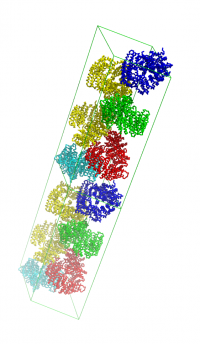# Difference between revisions of "Supercell"

Module Included in psico This command or function is available from psico, which is a PyMOL extension. psico.xtalExample with 2 unit cells in c-direction, created with: supercell 1,1,2,2x19

supercell can display multiple copies of the unit cell. Can also fill the unit cell (and its copies) with symmetry mates.

Requires numpy.

## Example

```run supercell.py
fetch 2x19, async=0
supercell 2,1,1, 2x19, green
supercell 1,1,2, 2x19, orange, name=super2
```

## The Code

```'''
(c) 2010 Thomas Holder

PyMOL python script (load with `run supercell.py`)
Usage: See "help supercell" and "help symexpcell"
'''

from pymol import cmd, cgo, xray
from math import cos, sin, radians, sqrt
import numpy

def cellbasis(angles, edges):
'''
For the unit cell with given angles and edge lengths calculate the basis
transformation (vectors) as a 4x4 numpy.array
'''
basis = numpy.identity(4)
basis = sqrt(1 - basis**2 - basis**2)
edges.append(1.0)
return basis * edges # numpy.array multiplication!

def supercell(a=1, b=1, c=1, object=None, color='blue', name='supercell', withmates=1):
'''
DESCRIPTION

Draw a supercell, as requested by Nicolas Bock on the pymol-users
mailing list (Subject: [PyMOL] feature request: supercell construction
Date: 04/12/2010 10:12:17 PM (Mon, 12 Apr 2010 14:12:17 -0600))

USAGE

supercell a, b, c [, object [, color [, name [, withmates]]]]

ARGUMENTS

a, b, c = integer: repeat cell in x,y,z direction a,b,c times
{default: 1,1,1}

object = string: name of object to take cell definition from

color = string: color of cell {default: blue}

name = string: name of the cgo object to create {default: supercell}

withmates = bool: also create symmetry mates in displayed cells
{default: 1}

show cell

'''
if object is None:
object = cmd.get_object_list()
withmates = int(withmates)

sym = cmd.get_symmetry(object)
cell_edges = sym[0:3]
cell_angles = sym[3:6]

basis = cellbasis(cell_angles, cell_edges)
assert isinstance(basis, numpy.ndarray)

ts = list()
for i in range(int(a)):
for j in range(int(b)):
for k in range(int(c)):
ts.append([i,j,k])

obj = [
cgo.BEGIN,
cgo.LINES,
cgo.COLOR,
]
obj.extend(cmd.get_color_tuple(color))

for t in ts:
shift = basis[0:3,0:3] * t
shift = shift[:,0] + shift[:,1] + shift[:,2]

for i in range(3):
vi = basis[0:3,i]
vj = [
numpy.array([0.,0.,0.]),
basis[0:3,(i+1)%3],
basis[0:3,(i+2)%3],
basis[0:3,(i+1)%3] + basis[0:3,(i+2)%3]
]
for j in range(4):
obj.append(cgo.VERTEX)
obj.extend((shift + vj[j]).tolist())
obj.append(cgo.VERTEX)
obj.extend((shift + vj[j] + vi).tolist())

if withmates:
symexpcell('m%d%d%d_' % tuple(t), object, *t)

obj.append(cgo.END)

cmd.delete(name)

def symexpcell(prefix='mate', object=None, a=0, b=0, c=0):
'''
DESCRIPTION

Creates all symmetry-related objects for the specified object that
occur with their bounding box center within the unit cell.

USAGE

symexpcell prefix, object, [a, b, c]

ARGUMENTS

prefix = string: prefix of new objects

object = string: object for which to create symmetry mates

a, b, c = integer: create neighboring cell {default: 0,0,0}

symexp, http://www.pymolwiki.org/index.php/SuperSym
'''
if object is None:
object = cmd.get_object_list()

sym = cmd.get_symmetry(object)
cell_edges = sym[0:3]
cell_angles = sym[3:6]
spacegroup = sym

basis = cellbasis(cell_angles, cell_edges)
basis = numpy.matrix(basis)

extent = cmd.get_extent(object)
center = sum(numpy.array(extent)) * 0.5
center = numpy.matrix(center.tolist() + [1.0]).T
center_cell = basis.I * center

extra_shift = [[float(i)] for i in (a,b,c)]

i = 0
matrices = xray.sg_sym_to_mat_list(spacegroup)
for mat in matrices:
i += 1

mat = numpy.matrix(mat)
shift = numpy.floor(mat * center_cell)
mat[0:3,3] -= shift[0:3,0]
mat[0:3,3] += extra_shift

mat = basis * mat * basis.I
mat_list = list(mat.flat)

name = '%s%d' % (prefix, i)
cmd.create(name, object)
cmd.transform_object(name, mat_list)
cmd.color(i+1, name)

cmd.extend('symexpcell', symexpcell)
cmd.extend('supercell', supercell)

# tab-completion of arguments
cmd.auto_arg['supercell'] = [ cmd.object_sc, 'object', '']
```